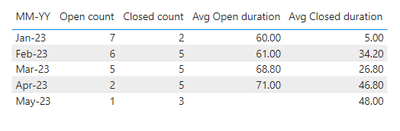cancel
Showing results for
Did you mean:Regular Visitor

## History Capture

Hello Friends,

I have open and closed incidents and I need history of an open incidents and closed incidents for example

Jan month I have 10 open incidents and 2 got closed in Jan and 5 got closed in Feb now on the chart for Jan month it should show 8 open count and 2 closed count and when we slide into Feb month, we have to consider 8 open counts from Jan and if there are 10 cases opened in Feb month then the count would need to be 8 (jan Open) + 10 (new Feb Open) = 18 (cases should be for Feb), this is how I am looking for, if anyone knows on how we can get this acheived in Power BI, please do let me know your thoughts and thanks for all your help in advance!!

Below is the sample data

 Incident Start date End date Days aging INC001 1/1/2023 1/3/2023 2 INC002 1/2/2023 1/10/2023 8 INC003 1/3/2023 2/10/2023 38 INC004 1/4/2023 2/8/2023 35 INC005 1/5/2023 2/20/2023 46 INC006 1/6/2023 2/25/2023 50 INC007 1/7/2023 3/2/2023 54 INC008 1/8/2023 4/3/2023 85 INC009 1/9/2023 5/1/2023 112 INC010 2/1/2023 2/3/2023 2 INC011 2/2/2023 3/2/2023 28 INC012 2/3/2023 3/5/2023 30 INC013 2/4/2023 4/2/2023 57 INC014 3/1/2023 3/5/2023 4 INC015 3/2/2023 3/20/2023 18 INC016 3/3/2023 4/15/2023 43 INC017 3/4/2023 4/20/2023 47 INC018 4/1/2023 4/3/2023 2 INC019 4/2/2023 5/2/2023 30 INC020 5/1/2023 5/3/2023 2 Inc021 5/4/2023

My expected result would be like shown below.

 open count Closed count Avg Open duration Avg Closed duration Jan-23 7 2 48 5 Feb-23 6 5 49 34 Mar-23 5 5 Apr-23 2 5 May-23 1 3
1 ACCEPTED SOLUTIONCommunity Support

Hi @avanti295 ,

``````Calendar =
CALENDAR (
EOMONTH ( MIN ( 'Table'[Start date] ), -1 ) + 1,
EOMONTH ( MAX ( 'Table'[End date] ), 0 )
),
"Year", YEAR ( [Date] ),
"Month", FORMAT ( [Date], "MMMM" ),
"MonthSort", MONTH ( [Date] ),
"MM-YY", FORMAT ( [Date], "MMM-YY" ),
"YearMonth",
YEAR ( [Date] ) * 100
+ MONTH ( [Date] )
)``````

Measure:

``````Open count =
CALCULATE (
COUNTROWS ( 'Table' ),
FILTER (
'Table',
AND (
'Table'[Start date] <= MAX ( 'Calendar'[Date] ),
OR (
'Table'[End date] > MAX ( 'Calendar'[Date] ),
'Table'[End date] = BLANK ()
)
)
)
)``````
``Closed count = CALCULATE(COUNTROWS('Table'),FILTER('Table','Table'[End date] in VALUES('Calendar'[Date])))``
``````Avg Open duration =
CALCULATE (
AVERAGE ( 'Table'[Days aging] ),
FILTER (
'Table',
AND (
'Table'[Start date] <= MAX ( 'Calendar'[Date] ),
OR (
'Table'[End date] > MAX ( 'Calendar'[Date] ),
'Table'[End date] = BLANK ()
)
)
)
)``````
``Avg Closed duration = CALCULATE(AVERAGE('Table'[Days aging]),FILTER('Table','Table'[End date] in VALUES('Calendar'[Date])))``

Result is as below.Best Regards,
Rico Zhou

If this post helps, then please consider Accept it as the solution to help the other members find it more quickly.Community Support

Hi @avanti295 ,

``````Calendar =
CALENDAR (
EOMONTH ( MIN ( 'Table'[Start date] ), -1 ) + 1,
EOMONTH ( MAX ( 'Table'[End date] ), 0 )
),
"Year", YEAR ( [Date] ),
"Month", FORMAT ( [Date], "MMMM" ),
"MonthSort", MONTH ( [Date] ),
"MM-YY", FORMAT ( [Date], "MMM-YY" ),
"YearMonth",
YEAR ( [Date] ) * 100
+ MONTH ( [Date] )
)``````

Measure:

``````Open count =
CALCULATE (
COUNTROWS ( 'Table' ),
FILTER (
'Table',
AND (
'Table'[Start date] <= MAX ( 'Calendar'[Date] ),
OR (
'Table'[End date] > MAX ( 'Calendar'[Date] ),
'Table'[End date] = BLANK ()
)
)
)
)``````
``Closed count = CALCULATE(COUNTROWS('Table'),FILTER('Table','Table'[End date] in VALUES('Calendar'[Date])))``
``````Avg Open duration =
CALCULATE (
AVERAGE ( 'Table'[Days aging] ),
FILTER (
'Table',
AND (
'Table'[Start date] <= MAX ( 'Calendar'[Date] ),
OR (
'Table'[End date] > MAX ( 'Calendar'[Date] ),
'Table'[End date] = BLANK ()
)
)
)
)``````
``Avg Closed duration = CALCULATE(AVERAGE('Table'[Days aging]),FILTER('Table','Table'[End date] in VALUES('Calendar'[Date])))``

Result is as below.Best Regards,
Rico Zhou

If this post helps, then please consider Accept it as the solution to help the other members find it more quickly.